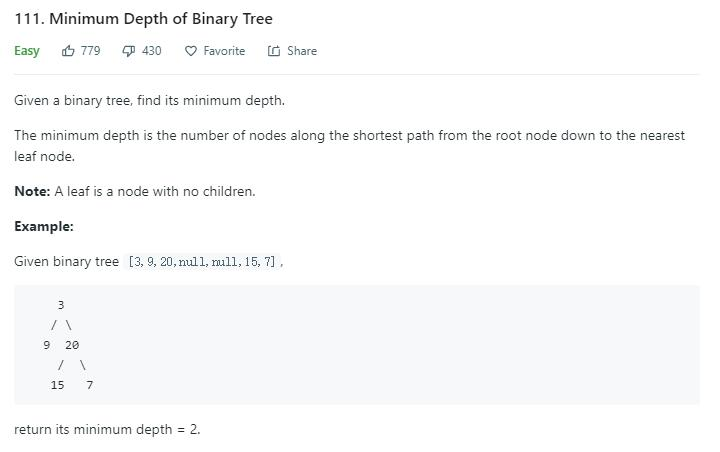# 题目描述（简单难度）# 解法一 递归

104 题 有些像，当时是返回根节点到叶子节点的最大深度。记得当时的代码很简单。

public int maxDepth(TreeNode root) {
if (root == null) {
return 0;
}
return Math.max(maxDepth(root.left), maxDepth(root.right)) + 1;
}


public int minDepth(TreeNode root) {
if (root == null) {
return 0;
}
return Math.min(minDepth(root.left), minDepth(root.right)) + 1;
}


     3
/ \
9   20
/  \
15   7


     3
/ \
9   20
/   /  \
8   15   7


public int minDepth(TreeNode root) {
if (root == null) {
return 0;
}
return minDepthHelper(root);

}

private int minDepthHelper(TreeNode root) {
//到达叶子节点就返回 1
if (root.left == null && root.right == null) {
return 1;
}
//左孩子为空，只考虑右孩子的方向
if (root.left == null) {
return minDepthHelper(root.right) + 1;
}
//右孩子为空，只考虑左孩子的方向
if (root.right == null) {
return minDepthHelper(root.left) + 1;
}
//既有左孩子又有右孩子，那么就选一个较小的
return Math.min(minDepthHelper(root.left), minDepthHelper(root.right)) + 1;
}


public int minDepth(TreeNode root) {
if (root == null){
return 0;
}
// 左孩子为空，只考虑右孩子的方向
if (root.left == null) {
return minDepth(root.right) + 1;
}
// 右孩子为空，只考虑左孩子的方向
if (root.right == null) {
return minDepth(root.left) + 1;
}
return Math.min(minDepth(root.left),minDepth(root.right)) + 1;
}


public int minDepth(TreeNode root) {
if (root == null) {
return 0;
}
if (root.left != null && root.right != null) {
return Math.min(minDepth(root.left), minDepth(root.right)) + 1;
} else {
return Math.max(minDepth(root.left), minDepth(root.right)) + 1;
}
}


# 解法二 BFS

104 题 也提供了BFS的方案，利用一个队列进行层次遍历，用一个 level 变量保存当前的深度，代码如下：

public int maxDepth(TreeNode root) {
if (root == null)
return 0;
queue.offer(root);
int level = 0;
while (!queue.isEmpty()) {
int levelNum = queue.size(); // 当前层元素的个数
for (int i = 0; i < levelNum; i++) {
TreeNode curNode = queue.poll();
if (curNode != null) {
if (curNode.left != null) {
queue.offer(curNode.left);
}
if (curNode.right != null) {
queue.offer(curNode.right);
}
}
}
level++;
}
return level;
}


public int minDepth(TreeNode root) {
if (root == null)
return 0;
queue.offer(root);
/**********修改的地方*****************/
int level = 1;
/***********************************/
while (!queue.isEmpty()) {
int levelNum = queue.size(); // 当前层元素的个数
for (int i = 0; i < levelNum; i++) {
TreeNode curNode = queue.poll();
if (curNode != null) {
/**********修改的地方*****************/
if (curNode.left == null && curNode.right == null) {
return level;
}
/***********************************/
if (curNode.left != null) {
queue.offer(curNode.left);
}
if (curNode.right != null) {
queue.offer(curNode.right);
}
}
}
level++;
}
return level;
}


# 总

104 题 题对比着考虑的话，只要找到这道题的不同之处，代码就很好写了。